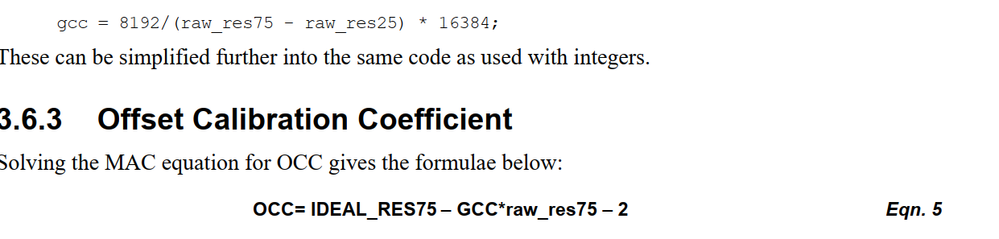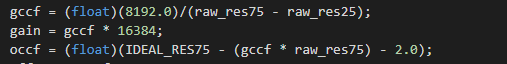cancel
Showing results for
Did you mean:
SOLVED

559 ViewsContributor III

Hello everyone,

There are two photos attached below. One of them is from an example whereas another is from a document about EQADC calibration.

In first photo, OCC was calculated by multiplying raw_res75 and GCC which was multiplyed with 16384 on the previous code line. However, in another photo, OCC was calculated without 16384. Which one is correct? Could you inform me?

Sincerely.1 Solution
543 ViewsNXP TechSupport

Calculation are based on following appnote:

https://www.nxp.com/docs/en/application-note/AN2989.pdf

GCC is an unsigned 15-bit fixed point value with a single-bit integer portion and a 14-bit fractional
portion.

OCC is a signed 14-bit integer value.

16384 = 0x4000, this is due to conversion from float to fractional format

544 ViewsNXP TechSupport

Calculation are based on following appnote:

https://www.nxp.com/docs/en/application-note/AN2989.pdf

GCC is an unsigned 15-bit fixed point value with a single-bit integer portion and a 14-bit fractional
portion.

OCC is a signed 14-bit integer value.

16384 = 0x4000, this is due to conversion from float to fractional format